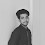# URI - BEECROWD - BEE 1040 | Average 3 Solution in C,C++,Python

## URI - BEECROWD - BEE Online Judge Solution  1040 | Average 3 - URI - BEECROWD - BEE 1040 Solution in C,C++,Python

Read four numbers (N1, N2, N3, N4), which one with 1 digit after the decimal point, corresponding to 4 scores obtained by a student. Calculate the average with weights 2, 3, 4 e 1 respectively, for these 4 scores and print the message "Media: " (Average), followed by the calculated result. If the average was 7.0 or more, print the message "Aluno aprovado." (Approved Student). If the average was less than 5.0, print the message: "Aluno reprovado." (Reproved Student). If the average was between 5.0 and 6.9, including these, the program must print the message "Aluno em exame." (In exam student).

In case of exam, read one more score. Print the message "Nota do exame: " (Exam score) followed by the typed score. Recalculate the average (sum the exam score with the previous calculated average and divide by 2) and print the message “Aluno aprovado.” (Approved student) in case of average 5.0 or more) or "Aluno reprovado." (Reproved student) in case of average 4.9 or less. For these 2 cases (approved or reproved after the exam) print the message "Media final: " (Final average) followed by the final average for this student in the last line.

## Input

The input contains four floating point numbers that represent the students' grades.

## Output

Print all the answers with one digit after the decimal point.

 Input Sample Output Sample 2.0 4.0 7.5 8.06.4 Media: 5.4Aluno em exame.Nota do exame: 6.4Aluno aprovado.Media final: 5.9
 2.0 6.5 4.0 9.0 Media: 4.8Aluno reprovado.
 9.0 4.0 8.5 9.0 Media: 7.3Aluno aprovado.

### URI Online Judge Solution  1040 | Average 3 - URI 1040 Solution in C,C++,Python :

URI Problem 1040 Solution in C :

 URI Online Judge 1040 Solve  in C :
```#include <stdio.h>
int main()
{
double first, second, third, fourth, last, sum;
scanf("%lf %lf %lf %lf", &first, &second, &third, &fourth);
sum = (first * 2 + second * 3 + third * 4 + fourth) / 10;
printf("Media: %.1f\n", sum);
if (sum >= 7.0){
}
else if (sum >= 5.0)
{
printf("Aluno em exame.\n");
scanf("%lf", &last);
printf("Nota do exame: %.1f\n", last);
if (last + sum / 2.0 > 5.0){
}
else{
}
printf("Media final: %.1f\n", (last + sum ) / 2.0);
}
else{
}
return 0;
}```

### 4 Responses to URI - BEECROWD - BEE 1040 | Average 3 Solution in C,C++,Python

1.why it is divided by 10

2.It is divided by 10 because total weight is 2+3+4+1 = 10 @walid

3.why you multiply the 2,3,4 and 1 with the numbers?

4.Because weights are 2, 3, 4 e 1 respectively